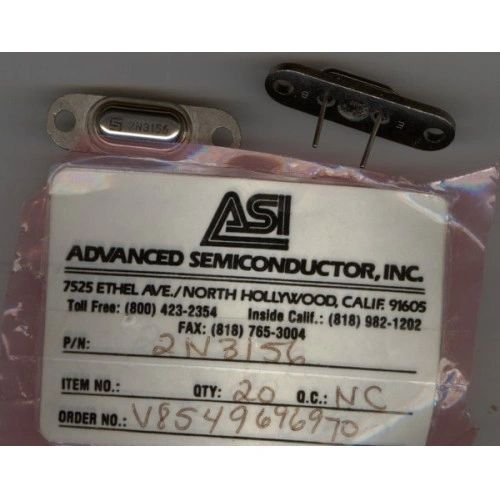•# TRANSISTOR 2N3156 ELECTRONICS PARTS

\$9.00 \$4.50
Free Shipping!

TRANSISTOR

2N3156

Part Number = 2N3156
Manufacturer Name = Various
Description = Ge PNP Power BJT
V(BR)CEO (V) = 55
V(BR)CBO (V) = 80
I(C) Abs.(A) Collector Current = 3.0
Absolute Max. Power Diss. (W) = 38
I(CBO) Max. (A) = 100u
h(FE) Min. Static Current Gain = 60
h(FE) Max. Current gain. = 180
@I(C) (A) (Test Condition) = 500m
@V(CE) (V) (Test Condition) = 2.0
f(T) Min. (Hz) Transition Freq = 15k
t(r) Max. (s) Rise time = 10u
Package = TO-3var
Military = N

NEW SURPLUS

NOTE:

ALL ELECTRONICS ARE UNTESTED

SOLD AS IS